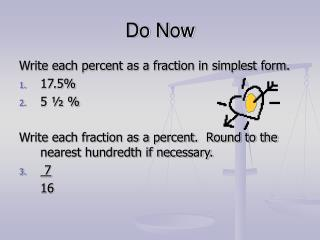DownloadDownload PresentationDo Now

# Do Now

Télécharger la présentation## Do Now

- - - - - - - - - - - - - - - - - - - - - - - - - - - E N D - - - - - - - - - - - - - - - - - - - - - - - - - - -
##### Presentation Transcript

1. Do Now Write each percent as a fraction in simplest form. • 17.5% • 5 ½ % Write each fraction as a percent. Round to the nearest hundredth if necessary. • 7 16

2. Chapter 7Lesson 6 Percents Greater than 100% and percents less than 1% Objective: Write percents greater than 100% and percents less than 1% as fractions and as decimals, and vise versa

3. Writing percents greater than 100% as fractions and as decimals. Method 1 (as a mixed number/fraction in simplest form) • Write the percent over 100. • Change to a mixed number. • Simplify Method 2 (as a decimal) 1. Move the decimal two places to the left.

4. Example 410% 410 100 410% 410 4.10 10 100 4 1 10 4

5. Write each percent as a decimal and as a mixed number or fraction in simplest form. • 325% 2. 475%

6. Writing percents less than 1% as fractions and as decimals. Method 1 (as a mixed number/fraction in simplest form) • Write the percent over 100. • Add the amount of zeros there are decimal places. • Simplify Method 2 (as a decimal) 1. Move the decimal two places to the left.

7. Example 0.2% 0.2 100 0.2% .002 2 1000 1 500

8. 1. 325% 3.25 3 ¼ 200% 2 2 Write each percent as a decimal and as a mixed number or fraction in simplest form.

9. 0.7% 0.007 7 1000 0.15% 0.0015 3 2,000 Write each percent as a decimal and as a mixed number or fraction in simplest form.

10. 1.8 180% 0.0015 0.15% Write each decimal as a percent.

11. Write each decimal as a percent. • 2.75 275% • 0.0005 0.05%

12. 9 ¾ % 975% 1 400 0.25% Write each number as a percent

13. Turn to page 318 in your textbooks Do numbers 19-35 odd